# How to Calculate and Solve for the Number of Entities of a Constituent, Volume and Number Concentration in Chemistry | Nickzom Calculator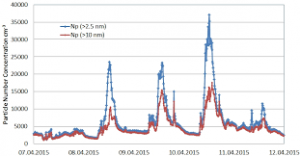The image above represents the number concentration.

To compute for the number concentration, two essential parameters are needed and these parameters are Number of Entities of the Constituent (N) and Volume (V).

The formula for calculating the number concentration:

C = NV

Where;
C = Number Concentration
N = Number of Entities of the Constituent
V = Volume

Let’s solve an example;
Find the number concentration when the number of entities of the constituent is 12 and the volume is 24.

This implies that;
N = Number of Entities of the Constituent = 12
V = Volume = 24

C = NV
C = 1224
C = 0.5

Therefore, the number concentration is 0.5 m-3.

Calculating the Number of Entities of the Constituent when Number Concentration and Volume is Given.

N = VC

Where;
N = Number of Entities of the Constituent
C = Number Concentration
V = Volume

Let’s solve an example;
Find the number of entities of the constituent when the number concentration is 18 and the volume is 14.

This implies that;
C = Number Concentration = 18
V = Volume = 14

N = VC
N = 14 x 18
N = 252

Therefore, the number of entities of the constituent is 252 m-1.

Calculating the Volume when Number Concentration and Number of Entities of the Constituent is Given.

V = N / C

Where;
V = Volume
N = Number of Entities of the Constituent
C = Number Concentration

Let’s solve an example;
Find the volume when the number concentration is 280 and the number of entities of the constituent is 40.

This implies that;
C = Number Concentration = 280
N = Number of Entities of the Constituent = 40

V = N / C
V = 280 / 40
V = 7

Therefore, the volume is 7 m3.

Nickzom Calculator – The Calculator Encyclopedia is capable of calculating the number concentration.

To get the answer and workings of the number concentration using the Nickzom Calculator – The Calculator Encyclopedia. First, you need to obtain the app.

You can get this app via any of these means:

To get access to the professional version via web, you need to register and subscribe for NGN 1,500 per annum to have utter access to all functionalities.
You can also try the demo version via https://www.nickzom.org/calculator

Once, you have obtained the calculator encyclopedia app, proceed to the Calculator Map, then click on Basic Chemistry under Chemistry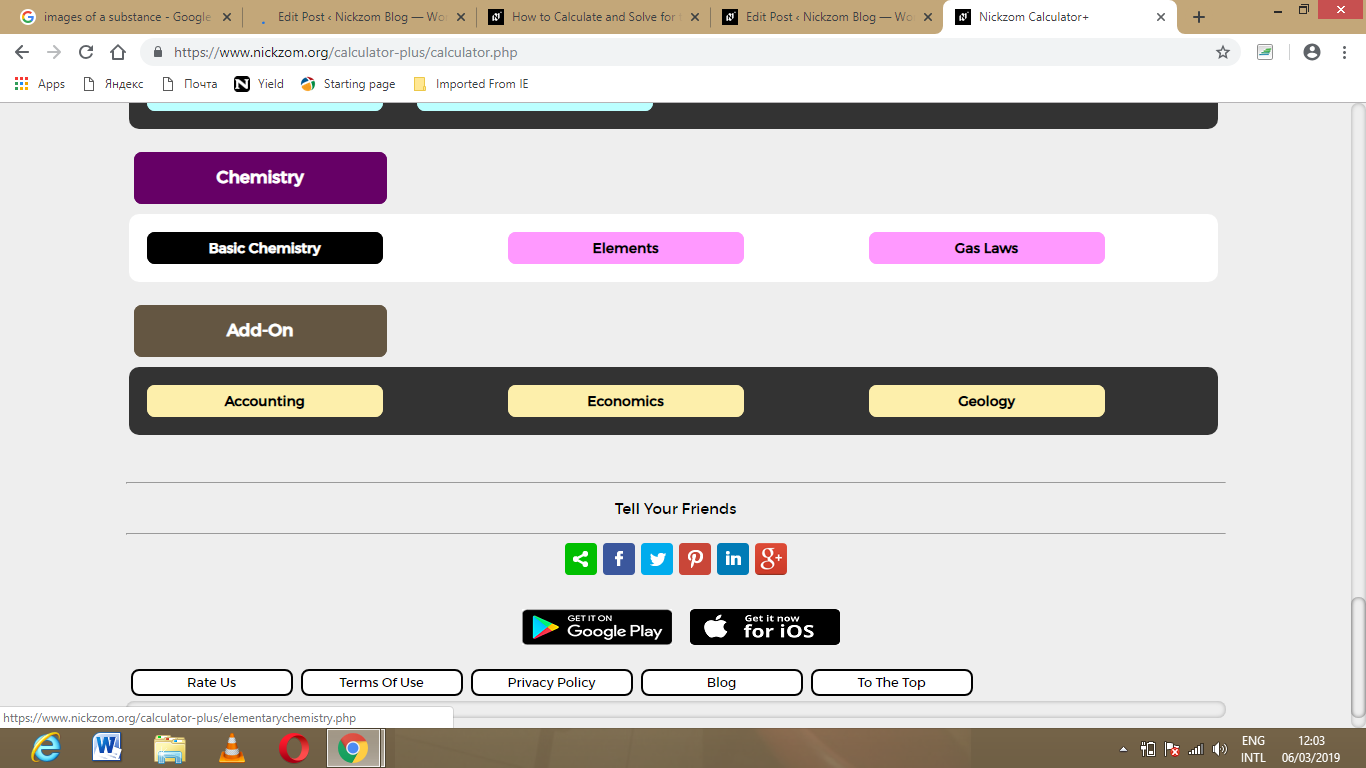Now, Click on Number Concentration under Basic Chemistry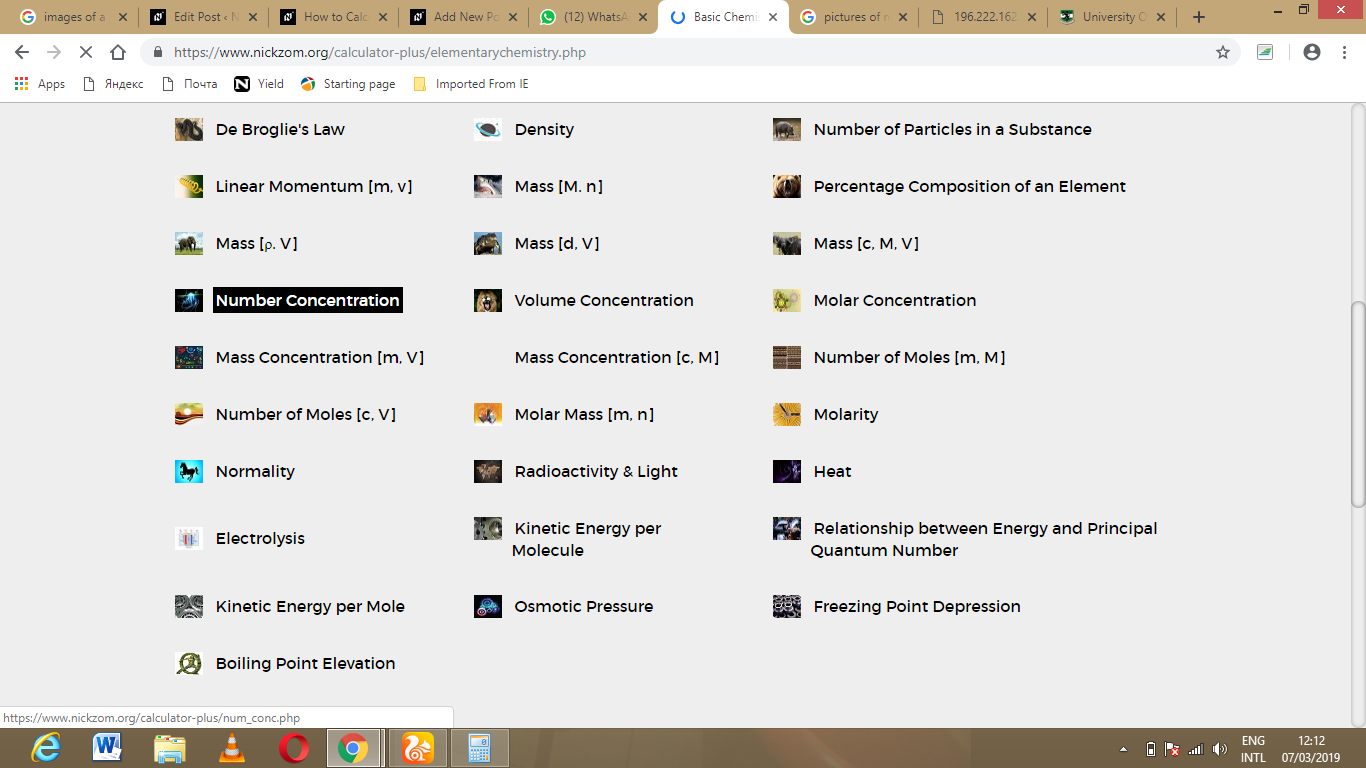The screenshot below displays the page or activity to enter your values, to get the answer for the number concentration according to the respective parameters which are the Number of Entities of the Constituent (N) and Volume (V).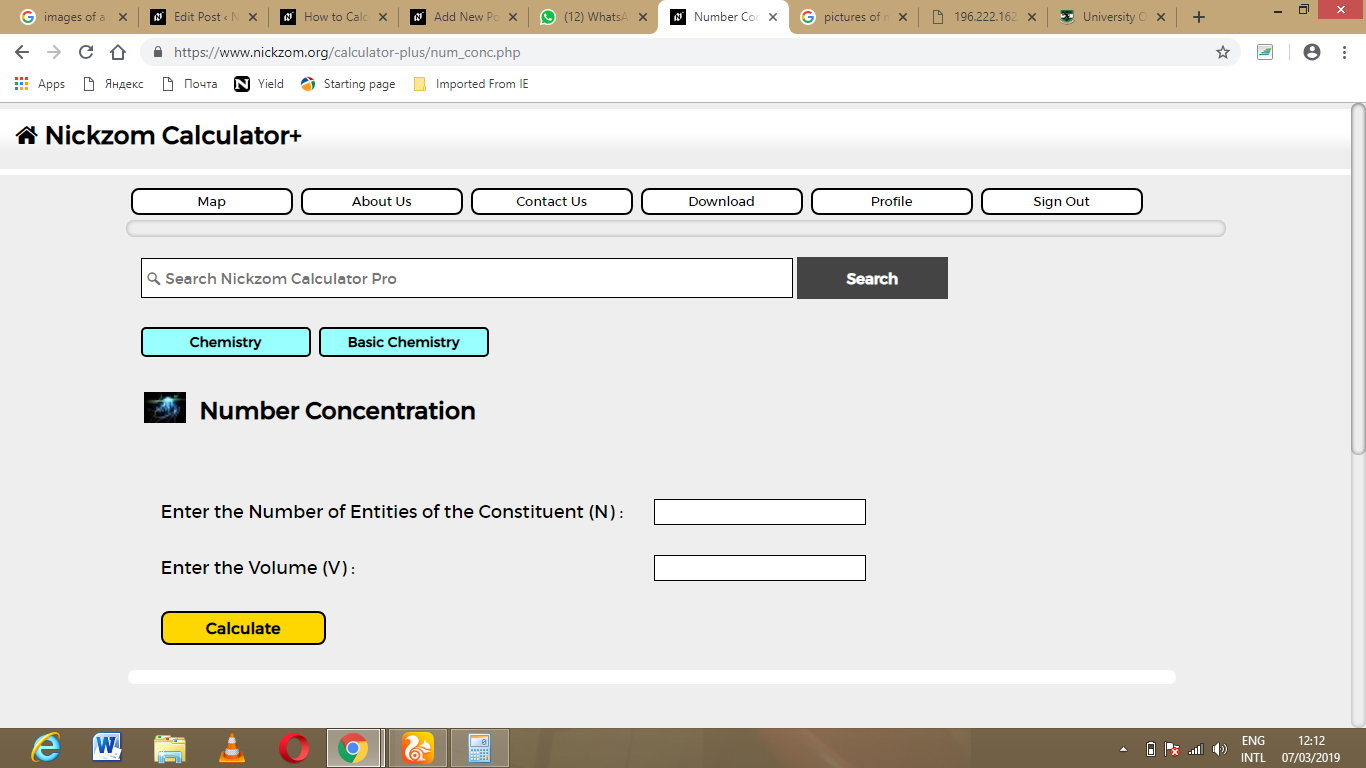Now, enter the values appropriately and accordingly for the parameters as required by the example above where the Number of Entities of the Constituent (N) is 12 and Volume (V) is 24.Finally, Click on Calculate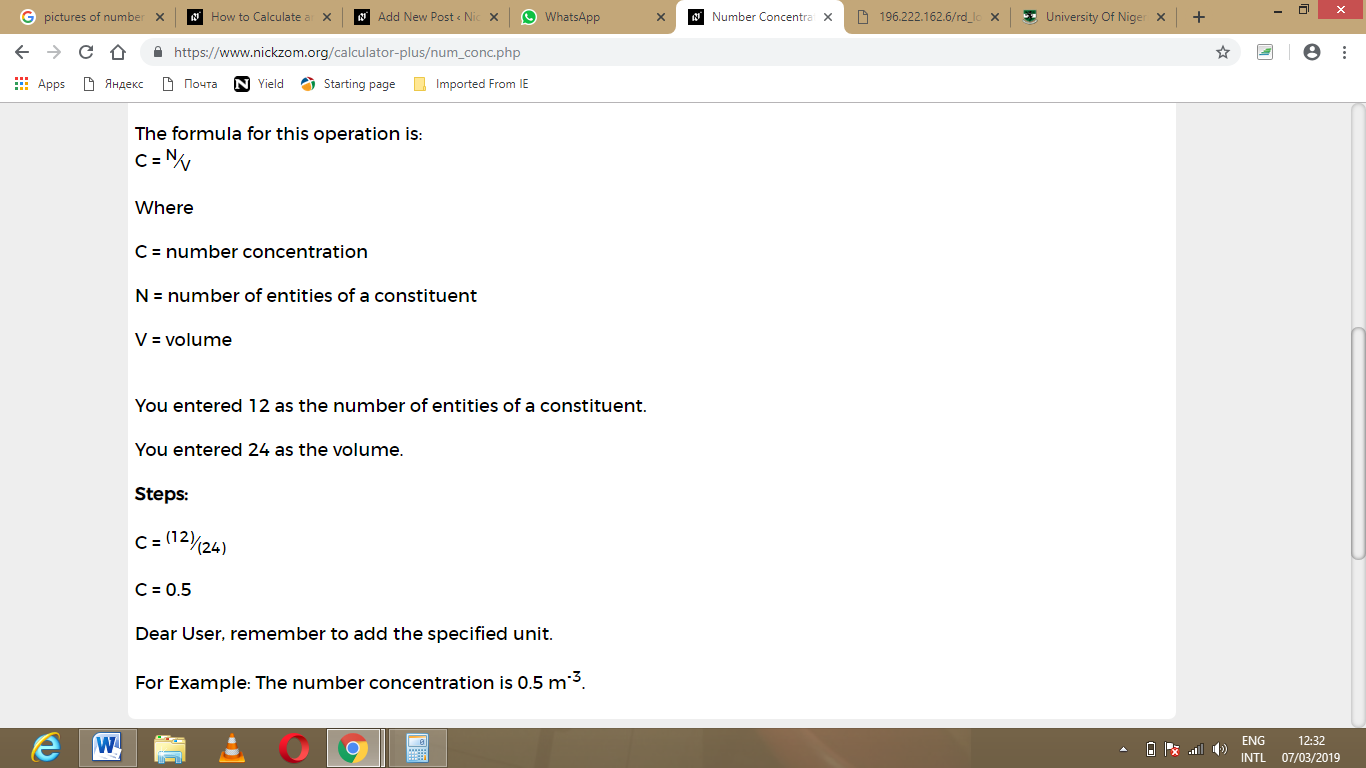As you can see from the screenshot above, Nickzom Calculator – The Calculator Encyclopedia solves for the number concentration and presents the formula, workings and steps too.